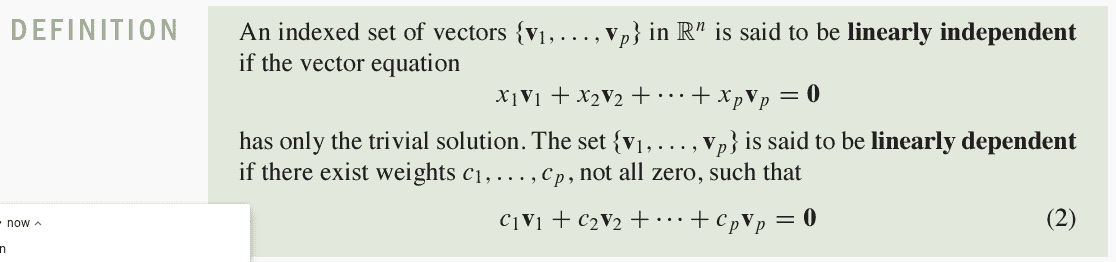# Q01 are linearly independent vectors, so are....

• MHB
Gold Member
MHB
Let A be invertible. Show that, if $\textbf{$v_i,v_2,v_j$}$ are linearly independent vectors, so are \textbf{$Av_1,Av_2,Av_3$}ok I think this is the the definition we need for this practice exam question,
However I tried to insert using a link but not successful
I thot if we use a link the image would always be there unless we delete its source

as to the question... not real sure of the answer since one $c_n$ may equal 0 and another may not

Anyway Mahalo...

You have "Show that, if $v_1$, $v_2$, $v_3$ are linearly independent, then so are $Av_1$, $Av_2$, $Av_3$" but what is "A"? If it is a general linear transformation this is not true. If A is an INVERTIBLE linear transformation then it is true and can be shown by applying $A^{-1}$ to both sides of$x_1Av_1+ x_2Av_2+ x_3Av_3= 0$.